Subhash Meena — Updated On August 19th, 2020

## Overview

• Get to know what the Monty Hall Problem is.
• Understand conditional probability with the use of Monty Hall Problem.

## Introduction

I was indulged in a project where we aim to predict the IPL auction prices for cricket players in such a manner that every franchise gets maximum of their choices in their team and every player gets an optimized price according to his caliber. Here we used rule-based classification which was based on “conditional probability”.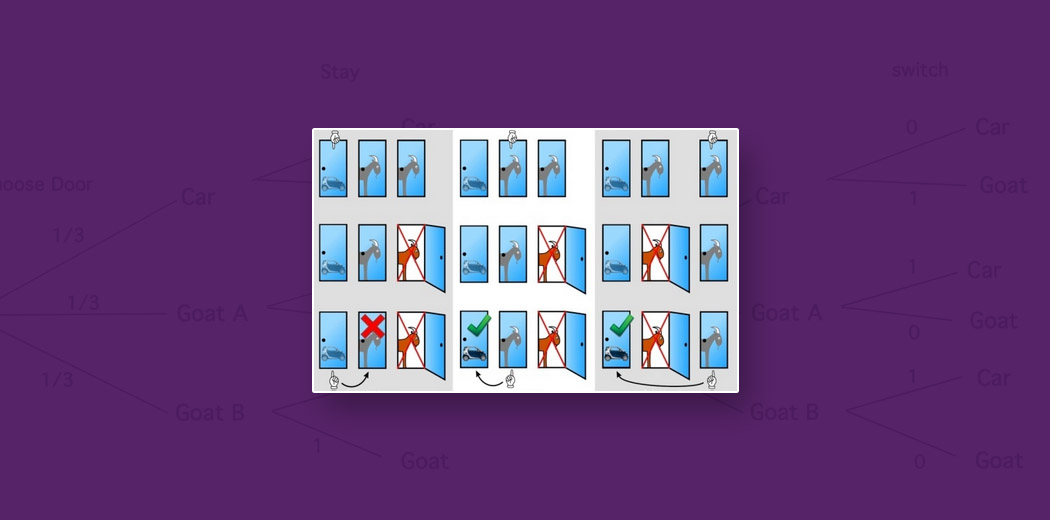Since then I started exploring conditional probability and a few days back I confronted a very interesting puzzle called “Monty Hall Problem”.The Monty Hall Problem became world-famous in 1990 when “Marilyn Vos Savant” gave a simple yet logical solution in the popular weekly column “Ask Marilyn” in Parade magazine as a reply to the following question-

Suppose you’re on a game show, and you’re given the choice of three doors: behind one door is a car; behind the others, goats. You pick a door, say No. 1, and the host, who knows what’s behind the doors, opens another door, say No. 3, which has a goat. He then says to you, “Do you want to pick door No. 2?” Is it to your advantage to switch your choice?”

And as usual, many statisticians taunted her saying that women don’t understand statistics but later all those critics ate humble pie and appreciated “Marilyn Vos Savant”. They all forgot the fact that at that time she was the person with the highest IQ as per the Guinness Book of Records

So in this article, I will explain to you the concept of conditional probability in detail using the Monty Hall Problem.

In case you want to learn these concepts and start your journey in data science, check out the following course- Introduction to Data Science

1. The Monty Hall Problem
2. Intuitive Explanation
3. Situational Explanation
4. Detailed Explanation
5. Explanation with Conditional Probability

## The Monty Hall Problem

Consider this scenario – Suppose you are in a game show and they give you three doors.

They have been caged.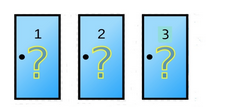Behind one door is a car and behind the other two doors are goats. (But you don’t know which door has what).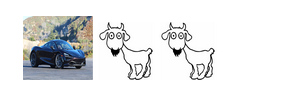Now the host of the game show asks you to pick one door. Note that he knows what is behind which door.Suppose you choose one of the doors among these three. Now, out of the remaining two doors,  the host reveals one door which has a goat behind it.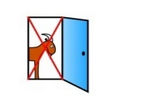And makes a very interesting proposal for you.

He asks you if you want to stick to the choice of your door or want to choose the door that has not been revealed yet?

What do you think? Do you want to switch the door as your choice or want to stick with your earlier choice? You must be thinking that it doesn’t matter. Because there are only 2 doors left and each of them has a 0.5 probability of having a car? Right?

But that’s not correct, let’s figure out how.

## Intuitive Explanation

Let’s think about it Cognitively first.

Let’s say that instead of three doors, you now have 1000 doors, of which only one has a car behind it while 999 will have a goat. You are asked to choose again, and you are select a door. The host then reveals 998 doors (of the remaining 999 doors) which have goats behind them. Two doors still remain closed – one of them is the one you picked previously. What would you do?

Do you want to switch now? Probably the answer is yes, but what is the reason for switching now?
Is it self realization or some cognitive thought occurring in the statistical mind.

The reason you want to switch now is that you have realized that probability of choosing the door which has the car at a first guess is 1/1000, So if you don’t switch, you only have 1/1000 chance of winning

## Situational Explanation

Have a look at the image below, which shows three possible scenarios. Suppose door 1 has a car behind it and the other two doors have goats.

• In the first case, you selected the first door
• While in the second case you selected the second door
• And in the third case, you selected the third door.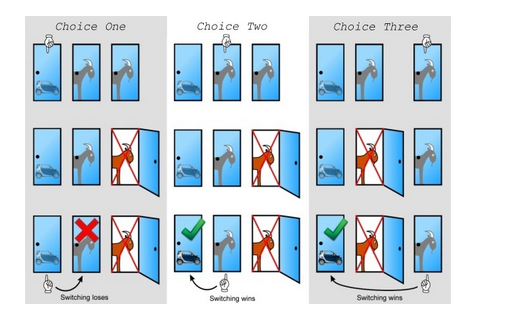Situation 1: You pick door one at your first guess. The host can reveal door 2 or 3 because both of them have goats. Suppose the host reveals door 3 which has a goat behind it, Now if you switch to door 2 you will lose.

Situation 2: What if you picked door 2 initially? Door 1 has a car so the host can not reveal it, he has to reveal door 3 which has a goat. Now if you switch to door 1 from door 2 you will win.

Situation 3: Similarly what if you picked door 3 initially? Again in this case, since door 1 has a car so the host can not reveal it, he has to reveal door 2 which has a goat. Now if you switch to door 1 from door 3 you will win again.

So in case of switching you are winning 2 out of 3 cases. So Probability of winning after the switch is ⅔ while the Probability of winning sticking with the initial choice is ⅓.

To summarise we can say that If you don’t switch, you will win the car only if you were correct in guessing right door initially Prob = ⅓ = 0.33
If you switch, you will win the car only if you were not correct in guessing the right door initially = ⅔ = 0.66.

## Detailed Explanation

Let’s understand this problem now with the concepts of Probability. A typical random experiment involves several randomly-determined quantities which are-

1. The door concealing the car.
2. Door that is initially chosen by the player.
3. The door that the host opens to reveal a goat.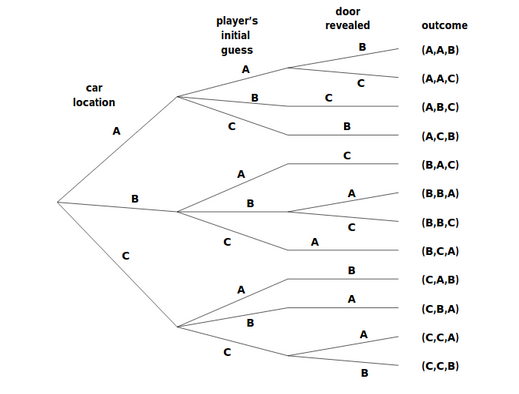As you can see above, this Tree Diagram represents all possibilities of this Puzzle. Car location, Player’s guess, and doors revealed making the outcome. All possible values of the outcome make the sample space. Let’s understand what each of these outcomes represents. For example, BAC here represents that car is located behind B, your initial guess is A and Host revealed the door C.

Out of this sample space, how will you define the events? For example, the event that the prize is behind door C is the set of outcomes:

{(C,A,B),(C,B,A),(C,C,A),(C,C,B)}

Because the first letter represents the Car Location in our Tree Diagram.

The event that you initially picked the door concealing the prize is the set of outcomes:

{(A,A,B),(A,A,C),(B,B,A),(B,B,C),(C,C,A),(C,C,B)

Because the first Letter represents the Car Location and the second Letter represents your choice which needs to be same for this event (initially picked the door concealing the prize), what we’re really after is the is the set of outcomes of event that the player wins by switching:

{(A,B,C),(A,C,B),(B,A,C),(B,C,A),(C,A,B),(C,B,A)}

Why? Let’s look at any of these outcomes,

CBA represents that C has the Car, B was picked by you, and A is revealed. So switching will lead you to C door from B door which actually has the Car. Hence, you win. Similarly, all these outcomes make you win in case of switching.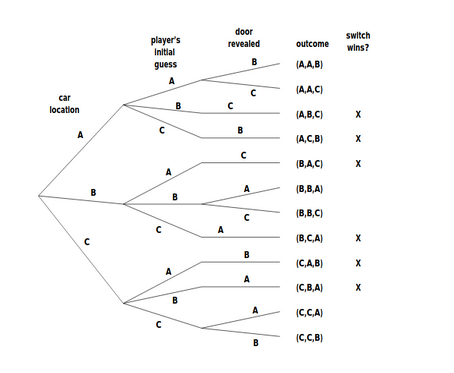Analyzing our Tree Diagram, we notice that exactly half of the outcomes are marked, meaning that the player wins by switching in half of all outcomes. You might be tempted to conclude that a player who switches wins with probability 1/2.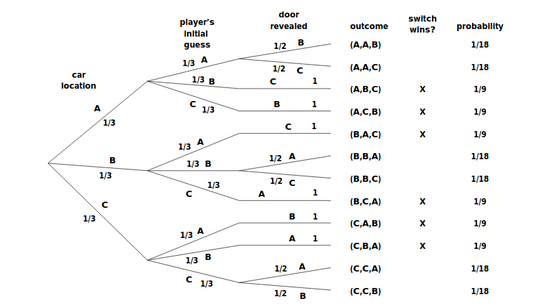This is wrong. The reason is that these outcomes are not equally likely. As you can see, outcome ABC and outcome BBA have different probabilities why?

#### Case 1

Let’s analyze, ABC first which means :

1. Car is behind Door A
2. You Picked Door B
3. And Host revealed Door C.

1. The car is behind door A,  has probability ⅓ because Car could be behind any of those three doors with equal probability.
2. You picking door B also has probability ⅓ because you could have picked any of the doors.
Thus this outcome has probability ⅓ * ⅓ = 1/9.
3. Now Host has door A and C, Host can not reveal door A because it has a car behind it so he must choose door C to reveal. Thus, the probability is 1 here.

Thus this outcome has probability ⅓ * ⅓ = 1/9.

#### Case 2

In Case of BBA, BBA  means :

1. Car is behind Door B
2. You Picked Door B
3. And Host revealed Door A

1. The car is behind door B,  has probability ⅓ because Car could be behind any of those three doors with equal probability.
2. You picking door B also has probability ⅓ because you could have picked any of the doors.
3. Now Host has doors A and C, Host can reveal any of the doors A and C because they both have a goat behind it so choosing door A by the host is Probability 1/2.

So this outcome has the Probability ⅓ * ⅓ * ½ = 1/18 as mentioned in the Tree Diagram. Thus Now it is clear that not all these outcomes are equally likely.

Let’s calculate the probability of winning in case of switching. Thus using the concepts of Sample Space, Event and Tree diagram

We conclude that:-

Probability (Switching wins)  = (P{A,B,C} + P{A,C,B} + P{B,A,C} + P{B,C,A} + P{C,A,B} + P{C,B,A})
= 1/9 + 1/9 + 1/9 + 1/9 +1/9 + 1/9 = ⅔

The reason why it is not ½ is because of the condition that every time after your pick host is revealing a door that has a goat behind it and this particular condition impacts the likelihood of final outcomes in our sample space.

## Explanation with Conditional Probability

The conditional probability is the probability of any event A given that another event B has already occurred. The idea here is that the probabilities of an event “maybe” affected by whether or not other events have occurred. The term “conditional” refers to the fact that we will have additional conditions, restrictions, or other information when we are asked to calculate this type of probability.

It is denoted in the following manner – “representing the Probability of A given B has occurred”

Conditional Probability can be calculated as Probability of A intersection B, divided by the probability of event B

P(A | B)  = P(A ∩ B) / P(B)

Let us start to analyze this problem when the contestant has chosen door 1. We assume that P(prize door i) = ⅓, for i = 1, 2, 3

If the prize is behind door 1 then the host show will open door 2 or door 3 each with probability 1/2.

So we have P(prize door 1 and host door 2) = 1/3 × 1/2 = 1/6

P(prize door 1 and host door 3) = 1/3 × 1/2 = 1/6

On the other hand, if the prize is behind door 2 or door 3, then the host has only one door that he can open, namely door 3 or door 2.

P(prize door 2 and host door 3) = 1/3 × 1 = 1/3

P(prize door 3 and host door 2) = 1/3 × 1 = 1/3

We have described all possibilities starting from the fact that the contestant has already chosen one door. Since the prize can be behind any door with the same probability, it does not matter which door is chosen. Given that the host opens door 3 the probability to win the prize by keeping the door is the conditional probability

P(Keep and win) = P(prize door 1 | host door 3)

= P(prize door 1 and host door 3)/ P( host door 3)

= (1/6) /P( host door 3)

while

P(Keep and loose) = P(prize door 2 | host door 3)

= P(prize door 2 and host door 3)/ P( host door3)

= 1/3 P/( host door 3)

It is therefore twice as likely to win by switching and so we have:-

P(Keep and win) = 1/3

P(Keep and loose) = ⅔

If one wishes to compute the probability that the host opens door 3 then one can find it by conditioning on the location of the prize:

P( host door 3) = P(host door 3| prize door 1)P(prize door 1)
+P(host door 3|prize door 2)P(prize door 2)
+P(host door 3|prize door 3)P(prize door 3)

= 1/2 × 1/3 + 1 × 1/3 + 0 × 1/3 = 1/2

With this, we conclude the Monty Hall Problem Explanation using Conditional Probability.

## End Notes

To summarize, in this article we explained the concept of conditional probability using the Monty Hall Problem. It is an imperative concept that all aspiring data scientists need to understand. Not only this, but there are also several other concepts that you should be well versed with. The following are some of the articles on statistics and probability that you should understand-

I hope this article was fruitful to you. Let us know in the comments in case you have any queries.•